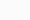# What Are Whole Numbers

## What Are Whole Numbers?

We see numbers everywhere around us in our daily life. Numbers are used for counting, measurement, telling time, and many other ways. Numbers are involved in almost everything. In mathematics, a whole number represents any positive number. However, it does not include a fractional or decimal number. Whole numbers are the set of basic counting numbers such as 0, 1, 2, 3, 4, .. and so on.

This means whole numbers start from 0 and then include all natural numbers from 1 onwards. In other words, whole numbers are a set of numbers including all positive integers and 0, but not any number less than zero. Thus, the smallest whole number is zero. So it can be said that whole numbers are a set of all-natural numbers along with zero (0). Zero is a number that is neither a positive number nor a negative number.

The primary difference between natural numbers and whole numbers is zero which is included in the set of the whole numbers but not in the set of natural numbers. Therefore, the set of whole numbers: W = {0,1,2,3,4,5,6,7,8,9,10…….}## Important Facts About Whole Numbers:

• All-natural numbers are whole numbers.
• All positive integers along with zero are whole numbers.
• The minimum whole number is zero.
• Whole numbers do not include fraction, decimal, and negative numbers
• One whole number is obtained by adding 1 to the previous whole number

## Properties of the Whole Number:

• Closure Property The sum and product of two whole numbers will always be a whole number. For example, 7 + 12 = 19 (whole number) and 5 × 8 = 40 (whole number) • Commutative Property The sum and the product of two whole numbers remain unchanged even after changing the order of the numbers.

For example, 4 + 9 = 9 + 4 =13 and 2 x 6 =6 x 2 =12 • Additive Identity When zero is added to a whole number, its value remains the same. For example, 24 + 0 = 24 • Multiplicative Identity When a whole number is multiplied by 1, its value remains the same. For example, 18 x 1 =18

## Natural Numbers

Natural numbers refer to all the whole numbers excluding zero (0). Natural numbers include all the positive integers starting from 1 that are used for counting purposes. Natural numbers do not include zero (0). Zero is not considered as a natural number because you can count natural numbers and we do not start from zero but 1. The smallest natural number is 1. Natural numbers are positive integers and include numbers from 1 to infinity.

These numbers are used for counting and calculation purposes. Therefore, the set of natural numbers: N = {1,2,3,4,5,6,7,8,9,10…….} Every natural number is a whole number because natural numbers are positive numbers starting from 1 till infinite.

Natural numbers are countable numbers because they do not include zero or negative numbers. They are the real numbers that include only the positive integers, but not zero, fractions, decimals, and negatives. So, it is understood that every natural number is a whole number and all whole numbers other is a natural number. except 0. We can say that the natural number is a subset of the whole numbers.

Related Post  Choosing the Perfect Marketing Agency## Important Facts about Natural Numbers:

• They are whole numbers (also called integers) with which we count.
• They are never less than zero which means they are always positive numbers
• Natural numbers do not include fractions and decimals
• Zero is not a natural number
• Natural numbers start from 1
• The next natural number is determined by adding 1 to the current natural number.
• Natural numbers have properties the same as whole numbers regarding addition and multiplication. These concepts can be learned in a fun way at cuemath.com.

khalida recently posted…Consumer expectations